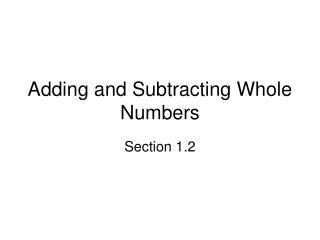DownloadDownload Presentation# Adding and Subtracting Whole Numbers

Télécharger la présentation## Adding and Subtracting Whole Numbers

- - - - - - - - - - - - - - - - - - - - - - - - - - - E N D - - - - - - - - - - - - - - - - - - - - - - - - - - -
##### Presentation Transcript

1. Adding and Subtracting Whole Numbers Section 1.2

2. Objectives • Find the sum of two or more whole numbers • Find the difference of two whole numbers • Estimate the sum or difference of whole numbers • Find the perimeter of a polygon

3. Objective 1:Find the sum of two or more whole numbers

4. Objective 1 • Addends are numbers that are added • Sum is the result of adding • To add whole numbers • Write numbers in columns so place values are lined up • Add each column, starting with the ones (or units) column • If sum of column is greater than 9, write the ones digit and carry the tens digit to next column

5. Objective 2:Find the difference of two whole numbers

6. Objective 2 • To subtract whole numbers • Write numbers in a column so place values are lined up • Subtract in each column, starting with one (or units) column • When numbers in a column can’t be subtracted, borrow 1 from next column and rename by adding 10 to upper digit, then subtract • When exercise is worded “Subtract A from B,” you must reverse the order: B – A.

7. Objective 3:Estimate the sum or difference of whole numbers

8. Objective 3 • Estimate by rounding numbers to a specified place and add or subtract

9. Objective 4:Find the perimeter of a polygon

10. Objective 4 • Polygon is a closed figure whose sides are line segments • Perimeter of a polygon is the distance around the outside • To find perimeter, add the lengths of the sides

11. Getting Ready for Algebra • Objective: Solve an equation of the form x + a = b, or x – a = b, where a, b, and x are whole numbers

12. Getting Ready for Algebra • Equation - statement about numbers that say two expressions are equal • Variables or Unknowns – letters that represent numbers • Solution – numbers that make equations true • Addition and subtraction are inverse operations

13. Getting Ready for Algebra • To solve an equation using addition or subtraction • Add the same number to each side of the equation to isolate variable, or • Subtract the same number to each side of the equation to isolate variable • Check the solution by substituting it for the variable in the original equation